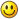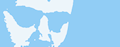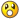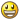## World Of Goo Screen Saver

48 replies [Last post]
Joined: 09/01/2009

That's the part I'm currently trying to think through. Using C++, I have a structure of strands that I'm hoping to be able to traverse to find a good location to build on. (Just in case you don't speak C++, a structure is a simple OOP data type) I'm not sure how to do this efficiently yet.
Of course, I don't have a lot of free time, so I'll probably either have to go for something simple or spend forever making it. I don't think I'm ready to launch into DirectX yet!Thanks for the pointers!

Edit: okay, I think I figured out a fairly ok way to do it. Start: an array of 100 aforementioned strand structures. Next, add an array of 100 attatched balls (100 is a reasonable maximum for this small-scale building, I think). The ball struct would contain pointers to up to six strands (the ones it is attatched to). If all the balls are updated correctly when they are attatched, adding another ball would be relatively easy. First, start stepping through the array of attatched balls, selecting two at a time. If two of their strand pointers match, they are connected and a ball can be built off of them (If one hasn't been already!). If their pointers don't match, they may need a strand between them, so the Pythagorean Theorem can be used to find the distance between them. If it is very close to the length of a strand, it must be a good spot. After this is determined, a non-attatched ball can be selected and dragged over to the "good" location. Because this would be random, the resulting structure would look really messy, but hopefully would work.

What do you think? Can you think of a better way? I'm open for options. This would be a quick-and-dirty solution, and would require a lot of calculations! If you think of a simpler way to do this, let me know, please!Joined: 11/04/2008

Given you're not worring about physics, you can do the following:

Start off with two balls, one strand. To the strand struct, add a "built on" flag, default false.

For each unattached ball, find a strand to build a triangle on. The candidate list is all strands where "built on" is false.

The ball's location, if built on a strand, is third corner of an equilateral triangle where the first two corners are the balls that strand attaches. The challenge is to select the best strand to build on from the candidate list. If you just choose the highest one, you'd end up with a unstable-looking structure going straight up into the air. If you choose one at random, you'll get very wonky looking structures since you're not simulating physics.

After choosing which strand to build on, move the ball, mark this strand as "built on", and add two new strands, one to each of the two existing balls.Joined: 10/16/2009

Whoa Davidc gave a WAY better explanation than I might have given.Joined: 11/04/2008

I tried to do a proof of concept for this but it's been too long since I've done any trigonometry!

Anyone any good at maths? Two circles of radius r centred about points A and B intersect at points M and N. What are the X and Y coordinates of M and N?

-davidc

Joined: 03/31/2009

WOW!!! That is exactly what I'm learning at the moment and have a exam on Saturday!!!(well i'm learning duble integrals but this also)

it's easy but a bit difficul to expalin but I'll try...
(sorry I don't know how to tipe sub and sup)

equation of the first circle is: sqr(X-X1)+sqr(Y-Y1)=sqr(r)
X - just x, Y - just y, X1,Y1 - coordinates, r - radius

equation of the second circle is: sqr(X-X2)+sqr(Y-Y2)=sqr(r)

from the firs you express X: X=sqrt(sqr(r)-sqr(Y-Y1))-X1

and the put it in the second equation, you get:
sqr((X=sqrt(sqr(r)-sqr(Y-Y1))-X1)-X2)+sqr(Y-Y2)=sqr(r)

from this you get the Y(unknown) as a number,
you put it in X=sqrt(sqr(r)-sqr(Y-Y1))-X1 equation
and from it you get X(unknown)
(you get two number for X and two for Y)

that's all. If I made a mistake it's probably a tipo!now the real calange is to calculate the length from M to N and surface of the second circle without the first and the volume it makes with "sqrX+sqrY+sqrZ=8" plane using "Green's equation"EDIT: you can use shifts: X=rcosα and Y=rsinα, and the calculate (r,α) of the M and N from the O( 0,0 ) (r-distance, α-angle from X)

My Gooish profile | Videos on YouTube | My WOG ModsJoined: 11/19/2009

davidc,

I made a spreadsheet that can find points M and N. Just enter the coordinates and radius for A and B in the highlighted boxes at the top. The formulas should be easily converted to whatever code you're using. Here's the link:

http://dl.dropbox.com/u/1422584/Goo.xls

Pavke, I think you might have a typo here:

Pavke wrote:
and the put it in the second equation, you get:
sqr((X=sqrt(sqr(r)-sqr(Y-Y1))-X1)-X2)+sqr(Y-Y2)=sqr(r)

Can that be simplified to solve for Y?

Do you use time flies when you're having fun?
http://jertech.blogspot.comJoined: 11/04/2008

Thanks guys... JerTech there's something not quite right with your spreadsheet, it looks like sometimes (but not always) the two X coordinates are the wrong way around? see attached graph:

http://goofans.com/sites/default/images/triangles%20with%20graph.xls

Joined: 03/31/2009

JerTech wrote:

Pavke, I think you might have a typo here:

Pavke wrote:
and the put it in the second equation, you get:
sqr((X=sqrt(sqr(r)-sqr(Y-Y1))-X1)-X2)+sqr(Y-Y2)=sqr(r)

Can that be simplified to solve for Y?

Yes it's a typo.it should be: sqr((sqrt(sqr(r)-sqr(Y-Y1))-X1)-X2)+sqr(Y-Y2)=sqr(r) without "X="

My Gooish profile | Videos on YouTube | My WOG ModsJoined: 11/19/2009

davidc,

You're correct. This happened when there was a negative slope. It should be fixed now. Try the link again.

Pavke,

Thought so, but are you still able to simplify that for Y?

Do you use time flies when you're having fun?
http://jertech.blogspot.com

Joined: 03/31/2009

Yes of course it can be for Y, it is only unknown it the equation, but back then I didn't have paper and pen, and now since I pass that exam I'm to lazy to do itMy Gooish profile | Videos on YouTube | My WOG Mods

Joined: 09/01/2009

Yeah, okay here. I understood Mr. Croft's first comment about making the strands have a "built on" flag. That's way better of an idea than mine. As for the other stuff... Well, let's just say that I'm still studying first integrals in Calculus this year, and I'm way behind you, Pavke. Anyway, I think I might have figured out locations to put the balls, but I'll have to tinker with it to get it right.

Pavke: if you or Mr. Croft could write what you said in C++ code for me, I could implement it with minimal brain stress on my part as possible. Thanks!

Edit: okay, I just thought of something. Since I'm using equilateral triangles with constant distances between them, I don't think I really need all the complicated code stuff. Also, I thought of a theoretical approach to building a tower that would look fairly good. It starts with two variables that keep track of the weight on either side of the tower, with an arbitrary line down the center that determines what is on the left side, and what is on the right. The closer to the bottom of the screen or the closer to the center line, the less mass is added to that particular side of the screen. Therefore, if a ball is built on the right side of the tower, the program logic would determine there is now more mass on the right side, and would build on the left side (of course, if both sides were equal, it could build on either side). If the code would avoid naturally high-mass-adding areas (to a certain extent), then a fairly stable-looking structure would be achieved. Am I making sense? Anyway, since the balls would be built on equilateral triangles, it would be impossible to build straight upwards, and I think this simulation would work.

The only other problem I can think of is determining when a strand needs to be built between two balls. Using Mr. Croft's (really good) idea for building, the checks for this would be a little far-fetched and would require some thoughtwork to implement. Any ideas?

Another thought: (boy, this is getting complicated) you would have to determine when it would be better to build a strand between two balls rather than building a ball onto a strand. For example:
o o
/\ /\
o-o-o
Kind of a not-very-good diagram, but anyway, if a ball was built on an inner strand, you would end up with two balls in one location (which I really want to avoid!), like so:
oo-o
/\\/\
o-o-o
With the two top left balls in the same position. A good algorithm for checking if a strand should be built, rather than a ball, would negate this problem entirely.

The only other thing I can think of at the moment is making sure a ball isn't built off the screen edge, but that is a fairly easy problem to solve.

Joined: 04/17/2009

Determining the weight of the two sides sounds like a good approach, but wouldn't that again lead to the problem that the tower is just built straight up?

my gooey profile | my video channel | author of Hazardous Environment

Joined: 03/31/2009

@MOM4Evr

sorry, i know C++ but don't know how to writh screensavers, maybe davidc can halp.
besides, you can just copy the equations abouve and set the starting X1, Y1, X2, Y2...

My Gooish profile | Videos on YouTube | My WOG Mods

Joined: 09/01/2009

thB: Yes, it would be built straight upwards, but in a more predictable fashion. (It wouldn't be built directly upwards in one thin line because that's impossible with equilateral triangles)

Pavke: Programming screensavers is 100% the same as making a normal Windows C++ program. The only difference is paying close attention to the command-line arguments. I discovered this by total accident.Joined: 11/19/2009

Pavke, if the equations above are not simplified, they cannot just be copied into code. You need to be able to solve for the new coordinates, so the equations must be simplified for those coordinates. For example, you don't want your code to say:

intVariableA = intVariableA * (other variables and operations)

In this case, that's not going to produce the result you want.

If someone wants to convert something into code, you can either try simplifying Pavke's equations or you can just use the formulae in the spreadsheet I created. The latter is going to be much easier. Just treat the various cells as variables, and you should be good to go.

Do you use time flies when you're having fun?
http://jertech.blogspot.com

Joined: 09/01/2009

Okay, right now I'm toying with just using constant locations for the distance from the other strands. This makes a uniform-looking structure without any glitches (so far!).

Anyway, right now I have a tower that builds up, then to the left, then up, then to the left, then up right off the screen. Once I make it able to build to the right, it will look a little better!Joined: 11/04/2008

Cool, the updated formula works. Here's a sample and source code: http://goofans.com/sites/default/towerbuilder/towerbuilder.html

I removed the "built on" flag since it's possible to build on either side of a strand. Instead, the candidate list is pruned to remove any ball location that is too close to an existing ball.

There's no logic to choose the "best" candidate at the moment: it just chooses the first available candidate, producing a nice snowflake patternIt could also use the option to place a ball between two existing balls to create a new strand by absorbing the ball, to bolster the structure.

The animation shows all candidate locations briefly in yellow, followed by the selected candidate in green.

-davidc

Joined: 09/01/2009

Okay, thanks! This should be a great help! (If I can figure out the Java!)

Edit: Figured out the Java. Now all I have to do is translate it into C++ for use in the screen saver. Thanks for the help, Mr. Croft!
(And could you code that strand-from-a-ball code idea for me?)(the "It could also use the option to place a ball between two existing balls to create a new strand by absorbing the ball, to bolster the structure" idea)